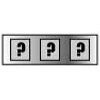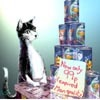#### You may also like### Forgotten Number

I have forgotten the number of the combination of the lock on my briefcase. I did have a method for remembering it...### Human Food

Sam displays cans in 3 triangular stacks. With the same number he could make one large triangular stack or stack them all in a square based pyramid. How many cans are there how were they arranged?### Hot Pursuit

I added together the first 'n' positive integers and found that my answer was a 3 digit number in which all the digits were the same...

# Sam Again

##### Age 11 to 14 Challenge Level:

You can make good use of a spreadsheet or of algebra to solve this problem. As well as solutions to Hannah's problem, solutions are invited to Santa's problem using methods which work equally as well for large numbers as for 21.

Congratulations to Jamesof Hethersett High School, Norfolk and to Helen, Charis, Lyndsay, Christiane, Charlotte, Bellaand Rachel, Year 10, Mount School York. Some said that either the reindeer were extremely hungry or there are more reindeer in Santa's employ than we are led to believe (someone did suggest 'Reindeer Express' along the lines of the Pony Express of history!) Here is Helen's solution:

To find how many layers high the pyramid is:

$$231 {\rm (cm)} / 11 {\rm (cm)} = 21 {\rm \ layers\ high.}$$

Each layer is a consecutive square number and the square numbers from $1^2$ to $21^2$ are:

1, 4, 9, 16. 25, 36, 49, 64, 81, 100, 121, 144, 169, 196, 225, 256, 289, 324, 361, 400, 441

All these added together gives 3311.

To find out how many cans in the triangular based pyramid, you add up the triangle numbers from the 1st to the 21st:

1, 3, 6, 10, 15, 21, 28, 36, 45, 55, 66, 78, 91, 105, 120, 136, 153, 171, 190, 210, 231

This equals 1771 cans.

The reindeer ate 3311 - 1771 = 1540 cans in one meal. There are 1771 cans left so there are enough to feed the reindeer another meal with 231 cans remaining.

James also solved Katherine's problem as follows. He wrote down all the triangular numbers smaller than 64 and then, using trial and improvement, James found two solutions:

$\begin{eqnarray} \\ T_5 + T_6 + T_7 &=& 15 + 21 + 28 = 64 \\ T_2 + T_3 + T_{10} &=& 3 + 6 + 55 = 64. \end{eqnarray}$

Well done Jacqui, Year 8, Mount School York for your solution to Nisha's problem. There are at least three ways of arranging 136 cans into four $T-$ stacks.

$\begin{eqnarray} \\ T_p + T_q + T_r + T_s &=& 136 \\ T_5 + T_6 + T_9 + T_{10} &=& 15 + 21 + 45 + 55 = 136 \\ T_3 + T_4 + T_5 + T_{14} &=& 6 + 10 + 15 + 105 = 136 \\ T_3 + T_7 + T_8 + T_{11} &=& 6 + 28+ 36 + 66 = 136. \end{eqnarray}$